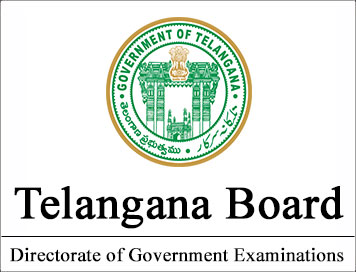# Telangana State Board CLASS-12 Model Question Papers - Chemistry Paper II

Disclaimer: This website is not at associated with CBSE, For official website of CBSE visit - www.cbse.nic.in

##### Year : 2013-14

BOARD OF INTERMEDIATE EDUCATION, A.P, HYDERABAD

MODEL QUESTION PAPER- CHEMISTRY II YEAR (W.E.F.2013-14)
Time: 3 Hours
Max.marks:60
SECTION-A
NOTE: Answer ALL the questions 10x2=20

1. What are Schottky defects in crystalline solids ?

2. Calculate the ‘spin only’ magnetic moment of Fe2+(aq) ion

3. Calculate the mole fraction of sodium hydroxide in 10% ww NaOH solution.

4. How is change in Gibbs energy (DG) related to the emf (E) of a galvanic cell?

5. What is the role of cryolite in the extraction of aluminium?

6. How do you distinguish between crystal lattice and unit cell?

7. What is PHBV? How is it useful to man?

8. Write the names and structures of the monomers of the following polymers
(a) Bakelite and (b) Nylon 6,6

9. Write the possible chain isomers of the compound having molecular formula C4H9Br.

10. How is ethane converted to bromoethane?

SECTION-B
NOTE: Answer any SIX of the following questions. 6x4=24

11. State Raoult’s law. Calculate the vapour pressure of a solution containing 9g of glucose in 162g of water at
293K. The vapour pressure of water at 293K is 17.535mm Hg.

12. What is catalysis ? What are the types of catalysis? Give one example for each type.

13. Differentiate roasting and calcination with examples.

14. Explain the structures of (a) XeF4 and (b) XeOF4

15. Explain Werner‘s theory of coordination compounds with suitable examples.

16. Give the sources of the following vitamins and name the diseases caused by their deficiency
(a) A (b) D (c ) E and (d) K

17. Write short notes on (a) Analgesics and (b) Food Preservatives

18. Explain (a) Sandmeyer reaction and (b) Carbylamine reaction.

SECTION-C
NOTE: Answer any TWO of the following questions. 2x8=16

19. (a) State and explain Kohlrausch’s law of independent migration of ions.

(b) What is order of a reaction? How is it different from the molecularity of a reaction? Give one example
each for first order and second order reactions

20. (a) How is ozone prepared? How does ozone react with
(i) PbS (ii) Moist KI (iii) Hg and (iv) Ag. Give equations.

(b) Write the balanced equations for the following
(i) NaCl is heated with conc. H2SO4 in the presence of MnO2
(ii) Chlorine is passed into a solution of NaI in water

21. (a) Explain (i) Cannizaro reaction and (ii) Decarboxylation

(b) Explain the acidic nature of phenols and compare it with that of alcohols.

*****